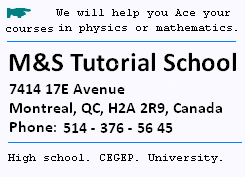Contents# Relativity: Momentum-Energy 4-Vector

### 1. Velocity 4-vector

We need to use the time derivative of the components of the 4-vector displacement. That is d(ct,x,y,z)/dt. We have with x = v t:

Vμ = d(ct, x)/dt = (c,v)
So
VμVμ = - c2 + v2,
which is not invariant. To become invariant, we use instead the proper time derivative. We recall that the proper time is the time in the rest frame of the particle; that is t' that we will denote by τ.
Then, with t = γ t'= γ τ:

Vμ = d(ct, x)/dt' = γ d(ct, x)/dt = γ (c, u)
So, dotting it into itself gives:
VμVμ = γ2 (- c2 + v2)
= - c2; which is invariant.

γ = 1/[1 - β2]1/2, where v is the velocity of the moving frame defined as x = vt from the rest frame.

VμVμ = - c2

The magnitude of the velocity 4-Vector is c, no matter the velocity v is.

Therefore:

Vμ = d(ct, x)/dt' = γ d(ct, x)/dt = γ (c, v)
is a velocity 4-Vector.

Vμ = γ (c, u) is a velocity 4-Vector

### 2. Relativistic energy

The relativistic energy E of the particle of mass at rest mo, then of mass m = γmo, when moving at v, is derived as:

m = γmo

dm/dv = mo dγ/dv

dγ/dv = d(1/[1 - β2]1/2)/dv =
v/c2 [1 - (v/c)2]-3/2 = (v/c2) (γ3)

Therefore:
dm/dv = mo (v/c2) (γ3) = m (v/c2) (γ2) =
m v/ (c2 - v2)

The force acting on the particle is F = dP/dt
F = dP/dt = d(mv)/dt = m dv/dt + v dm/dt =

We have:
dv/dt = (dv/dm)(dm/dt) = [(c2 - v2)/mv](dm/dt) = [(c2 - v2)/mv](dm/dt)

So
F = [(c2 - v2)/v](dm/dt) + v dm/dt = (c2/v)dm/dt

The energy of the particle as the received work:

dE = F dx = c2dm

Integrating gives:

E = c2m + constant.

If there is no mass then there is no energy. Hence Constant = 0; and we find again the equivalence mass-energy formula:
E = c2m

E = m c2

### 3. Momentum 4-Vector

According to the result from the velocity -4Vector, we define the momentum 4-Vector as the product of the velocity 4-Vector and the mass at rest mo of the particle moving at v from the frame at rest:

Pμ = mo Vμ = mo γ (c, v)

It is still a 4-Vector because we have multiplied by a constant.

Pμ is a 4-Vector.

We write:
m = mo γ , and then:

Pμ = m(c, v)

Pμ = m (c, v) = γ mo(c,v)

According to the result for the energy: E = m c2, we write:

Pμ = m (c, v) = (mc, mv) = (mc, p) = (mc2/c , p) = (E/c,p)

Pμ = (E/c,p)

Pμ = (E/c,p)

VμVμ = - c2 is the velocity 4-Vector Lorentz-invariant.
PμPμ = - mo2c2 is the momentum 4-Vector Lorentz-invariant.

Therefore, the Lorentz-invariant of the moment becomes:

= - (E/c)2 + p2 = - mo2c2, or
- E2 + p2c2 = - mo2c4

That is:

E2 = p2c2+ mo2c4

E2 = p2c2+ mo2c4

 chimie labs | Physics and Measurements | Probability & Statistics | Combinatorics - Probability | Chimie | Optics | contact |